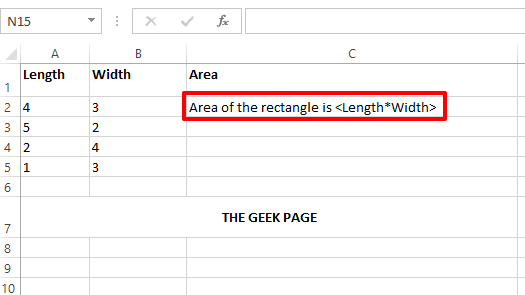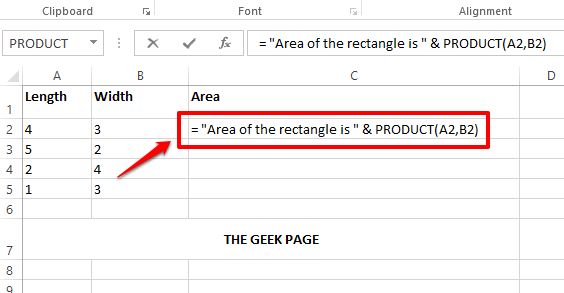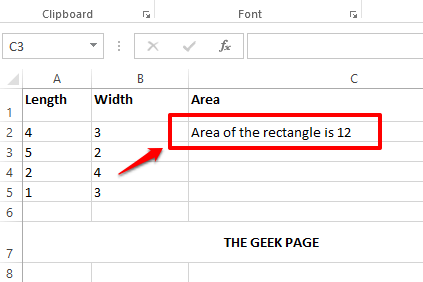# How to Add Text and Formula Inside a Cell in Microsoft Excel

Adding a formula to a cell in Excel is easy. Adding a text is even easier. But how about adding a text and a formula inside one single cell at the same time? Have tried but failed? Well, not anymore, when Geek Page is here to help you.

In this article, we explain in detail with the help of multiple examples how you can easily add a text and a formula both to one single cell in your Excel sheet. You can make your content dynamic, thus various requirements of yours regarding this can be easily met following the steps detailed in our article. Hope you enjoy!

## Example Scenario

Let’s say you have the following Excel table with the Length column and the Width column. You need to populate the Area column with the text Area of the rectangle is <Length*Width>. Where <Length*Width> should be replaced with the actual product of length and width to calculate the area.This is just an example scenario. You can have any text and any formula inside a cell in your Excel sheet, following the solution detailed here.

## Solution

Step 1: Firstly, type an = sign. Then if you are typing a text, include it in double quotes. To concatenate a formula to it, or add a variable in between, or add anything dynamic, use the concatenation operator &.

For example, in the example scenario mentioned in this article, you need to have the text Area of the rectangle is to come first. So, you need to double click your cell and write the content as = “Area of the rectangle is”. We have included this part inside double quotes because it is a text.

Now we need to calculate the area and append it to the text. So, put the concatenation operator & next. Then write the formula PRODUCT(A2, B2). The PRODUCT formula would calculate the product of the given 2 cell values.

So your final cell content for this particular case would look as follows.

`="Area of the rectangle is " & PRODUCT(A2,B2)`

Note: Dynamic means something that changes its value. For example, Area changes based on length and width. No double quotes for dynamic content. Static means something that doesn’t change its value. For example, text contents are static and they are to be put inside double quotes.Step 2: Once you have the cell contents all formatted, press the Enter key and you can see that the area of the rectangle is correctly calculated and that the value is successfully replaced.Step 3: You can click on the cell and then click and drag down the small square shape at the bottom right corner of the cell to apply the same formula and text to all the cells in the same column.Step 4: Below is a screenshot showing how the formula is applied to the entire column.Step 5: Now let’s make your cell a little more dynamic. Let’s say you want the text in your cell to be of the format Area of the rectangle with Length = <Length> and Width = <Width> is <Area>. Here, we have 3 values to be replaced. 2 variables, that is length and width, and one formula, which is the product of length and width. In this case, your content inside the cell should be as follows.

`="Area of the rectangle with Length= " & A2 & " and Width = " & B2 & " is " & PRODUCT(A2,B2)`

Explanation

1. First I have put the = operator.
2. Then enclosed the text content inside double quotes. That is, Area of the rectangle with Length = is enclosed in double quotes.
3. Next, I have put an & character to concatenate dynamic content.
4. Then the dynamic content is included by giving the cell id, which is length of the rectangle.
5. Next, I need to put an & again, because I’m going to append a text.
6. Then the text is written and enclosed in double quotes once again. The text this time is, ” and Width = “.
7. Again, & B2 & is put, to dynamically include the width into our cell value.
8. The last text we have in our cell is ” is “.
9. Finally, we need to append the PRODUCT formula, for which you need to put one last &.
10. Then the formula is written, PRODUCT(A2,B2).

Following the steps above, you can form any dynamic content inside your cell, as simple as that. Just remember one golden rule. Everything dynamic needs to be concatenated using & character without double quotes and everything static needs to be put inside double quotes.Step 6: If you press the Enter key, you can see that all the values are dynamically replaced and you can drag and apply the same format to all the cells within the same column as detailed in Step 3 too.If you are stuck at any of the steps, please drop us a comment, we would be happy to help. Stay tuned for more amazing tricks and tips.